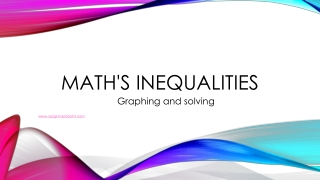DownloadDownload PresentationMath's Inequalities

# Math's Inequalities

Download Presentation## Math's Inequalities

- - - - - - - - - - - - - - - - - - - - - - - - - - - E N D - - - - - - - - - - - - - - - - - - - - - - - - - - -
##### Presentation Transcript

1. Math's Inequalities Graphing and solving www.assignmentpoint.com

2. Inequality Symbols Less Than < > < > = ≠ Greater Than Less Than or Equal To Greater Than or Equal To Equal To Not Equal To www.assignmentpoint.com

3. Graphing Symbols and Tools PEN CIRCLE – is used for <, >, and ≠. This means the number is not included in the solution. (where it starts) CLOSED CIRCLE – is used for <, >, and =. This means the number is included in the soluteon. (where it starts) Number Line – used for graphing solutions to inequalities www.assignmentpoint.com

4. How to Graph 1 2 3 4 5 6 7 1 2 3 4 5 6 7 1 2 3 4 5 6 7 x > 5 Where does it start? What type of circle will be used? Which direction will the arrow go? x < 3 Where does it start? What type of circle will be used? Which direction will the arrow go? x ≠ 4 Where does it start? What type of circle will be used? Which direction will the arrow go? www.assignmentpoint.com

5. Now you graph these x < 10 y > 4 z = 5 a ≠ 6 b ≠ - 8 c < -2 d > -3 www.assignmentpoint.com

6. Rule when solving inequalities Whendividing or multiplying by anegative number flip the sign. Example: - 5x > - 25 - 5x > - 25 - 5 - 5 x < 5 www.assignmentpoint.com

7. Solving one step inequalities c + 7 > 16 given - 7 -7 opposite of addition is subtraction c > 9 solution now we can graph it d + -9 < 20 given +9 +9 opposite of negative is positive d < 29 solution now we can graph it 8r > 64 ÷8 ÷8 r > 8 -5r > 45 ÷-5 ÷-5 r < -9 s÷12 > 4 ×12 ×12 s > 48 w÷-6 > -3 ×6 ×6 w < -18 www.assignmentpoint.com

8. You solve and Graph these one step inequalities (show your work) -56 + x > 100 x – 10 < 20 5p < - 30 -7q > -49 h ÷ -4 < -12 m ÷ 6 > -3 x > 156 x < 30 p < -6 q > -7 h < 48 m > -18 www.assignmentpoint.com

9. Solving two step inequalities x + 6 > -6 given 3 3(x + 6)> (-6) 3 opposite of divide is multiply 3 x + 6 > -18 still not done x + 6 – 6 > -18 – 6 now opposite of addition is subtraction x > -24 now graph the solution -5y – (-15) < -30 given -5y + 15 < -30 change subtraction to addition -5y + 15 – 15 < -30 – 15 opposite of addition is subtraction -5y < -45 not done yet -5y ÷ -5 < -45 ÷ -5 opposite of multiply is divide y > 9 have to flip sign and now we have the solution to graph 3x + 7 < 16 given -7 -7 opposite of addition is subtraction 3x < 9 still not done ÷3 ÷3 opposite of multiply is divide x < 3 solution now we can graph www.assignmentpoint.com

10. Now you solve and Graph these two step inequalities x + 6 > -6 -3x + 7 ≠ -26 -10x – (-10) < 100 y – (-7) < 15 3 -2 x > -24 x ≠ 11 x < 11 y < -37 www.assignmentpoint.com